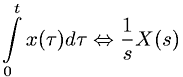Equations > Signal Processing > Laplace Transform Properties > Laplace transform integral property

### Laplace transform integral propertyLatex Code:

MathML Code:

 $\underset{0}{\overset{t}{\int }}x\left(\tau \right)d\tau ⇔\frac1sX\left(s\right)$

MathType 5.0: